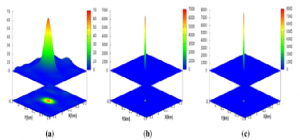# Statistical synthesis of phase alignment algorithms for localization of wave field sources

A article Statistical synthesis of phase alignment algorithms for localization of wave field sources has been published in the journal Multidimensional Systems and Signal Processing (https://doi.org/10.1007/s11045-020-00722-3). Authors: senior researcher, Ph. D. A. V. Varypaev and leading researcher, Ph. D. A. F. Kushnir.

This article is devoted to the problem of determining the coordinates of wave field sources using phases of complex Fourier transforms of signals recorded by the sensor array. Phasebased source localization algorithms have an important reliability property: they provide the quality of determining the source coordinates, which weakly depends on the statistical characteristics of random noise affecting the array sensors. For this reason, phase algorithms are widely used to localize wave radiation sources in radio and acoustic applications and, more recently, in seismology. Naturally, preference should be given to phase-based source localization algorithms that provide the smallest average errors in estimating source coordinates. Such algorithms can be constructed and analyzed using methods of mathematical statistics. In this article, we synthesized a phase-based statistically optimal (PSO) algorithm, which is a modification of the asymptotically efficient (ASE) statistical algorithm for estimating the coordinates of microseismic sources proposed in Kushnir et al. (Int J Geomath 4(2):201–225, 2013. https://doi.org/10.1007/s13137-013-0049-6). Unlike the ASE algorithm, the PSO algorithm does not require additional observations of “pure” noise and is resistant to the statistical properties of random noise. The article shows that the PSO algorithm is an extended version of the well-known phase-based SRP-PHAT algorithm (Brandstein and Ward in Microphone arrays signal processing techniques and applications, Springer, Berlin, 2001, Chapter 8; Zhang et al. in IEEE Trans Multimedia 10(3):538–548, 2008), which is widely used in acoustic applications. In this article, we describe Monte-Carlo simulation, the purpose of which is to compare the accuracy of determining the coordinates of micro-seismic sources provided by various phase-based algorithms. This simulation showed that the proposed PSO algorithm provides much better source positioning accuracy than the traditional SRP-PHAT algorithm. It is also shown that the PSO algorithm provides the source positioning accuracy, which is almost the same as for the phase-based source location algorithm recently synthesized by the maximum likelihood (ML) method in Varypaev and Kushnir (Int J Geomath 9(2):335–358, 2018. https://doi.org/10.1007/s13137-018-0108-0). However, the PSO algorithm has much higher computational efficiency than the ML algorithm, which allows it to be used for online processing of array data.Fig.Empirical probability distribution densities for estimates of the horizontal coordinates of micro-seismic source in the case of ASNR=0.3: a. SRP-PHAT algorithm; b. PML algorithm; c. PSO algorithm

Source: Varypaev A., Kushnir A. Statistical synthesis of phase alignment algorithms for localization of wave field sources // Multidimensional Systems and Signal Processing. 2020. DOI:10.1007/s11045-020-00722-3